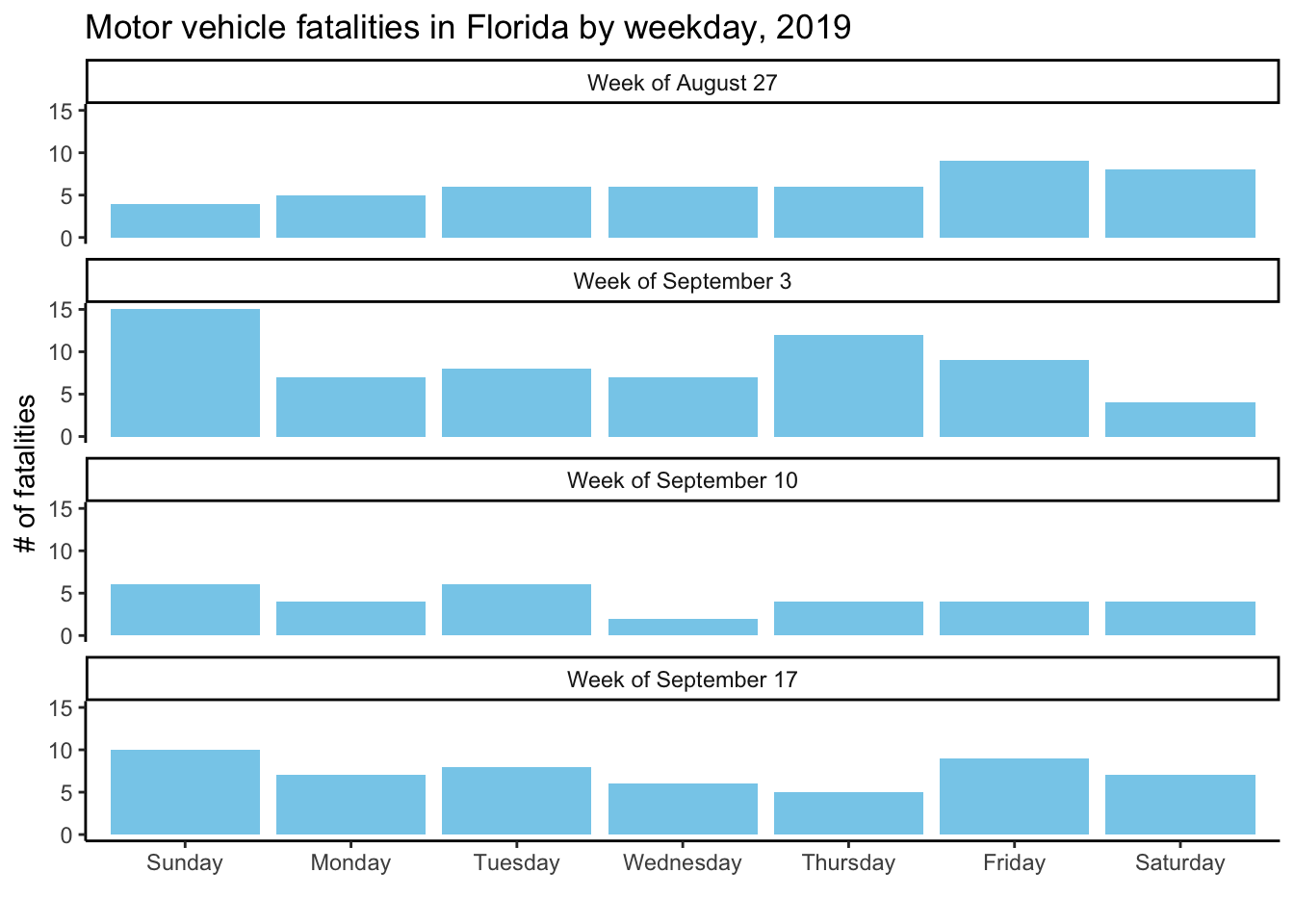## 2.3 Saving plots

You can assign the output of a `ggplot` call to an R object using the gets arrow (`<-`), just like you can assign data you’ve read in to an R object. If you do this, the plot won’t print immediately after you run the code:

``````irma_fatalities_plot <- ggplot(data = daily_fatalities) +
geom_col(aes(x = weekday, y = fatals), fill = "skyblue") +
facet_wrap(~ week_label, ncol = 1) +
labs(x = "", y = "# of fatalities") +
theme_classic() +
ggtitle("Motor vehicle fatalities in Florida by weekday, 2019")``````

However, now you can print the plot anytime you want by calling the object:

``irma_fatalities_plot``To save this plot to a file, you can use the `ggsave` function. This function can save the plot in several different formats (e.g., pdf, jpeg, png, svg). Further, it allows you to specify the size you’d like for the height and width of the plot as well as the plot resolution.

The following call will save the `irma_fatalities_plot` to your working directory, which should be the R project directory you’re using for the examples if you followed the set-up in “Prerequisites”. It will save the file as a pdf that is 6 inches tall by 5 inches wide.

``````ggsave(irma_fatalities_plot,
filename = "irma_fatalities.pdf",
device = "pdf",
height = 6, width = 5, units = "in")``````